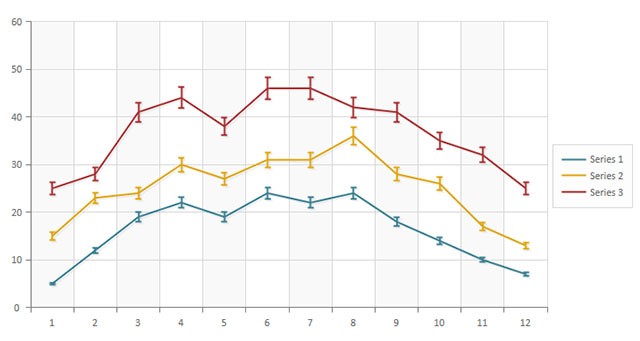# Chart Error BarsBCGControlBar Pro (MFC)BCGSuite (MFC)BCGControlBar for .NET

Chart Error Bars are implemented in the following chart categories: bar/column, line, area and polar/radar charts.

Error bars support the following types of errors for all chart types (simple, stacked, 100% stacked):

1. EBT_SEM_SE - Standard error of the mean based on a sample of the population
2. EBT_SEM_SD - Standard error of the mean based on the entire population
3. EBT_STD_S - Standard deviation based on a sample of the population (corrected sample standard deviation)
4. EBT_STD_P - Standard deviation based on the entire population
5. EBT_FIXED - Fixed value for each data point
6. EBT_PERCENT - Fixed percent for each data point
7. EBT_DIFF - Difference between previous and current data points
8. EBT_CUSTOM - The framework calls the BCGPCHART_ERRORBARS_CALLBACK function to calculate the values for each data point